# Probabilistic reasoning over time Ch 15 17 Probabilistic

• Slides: 27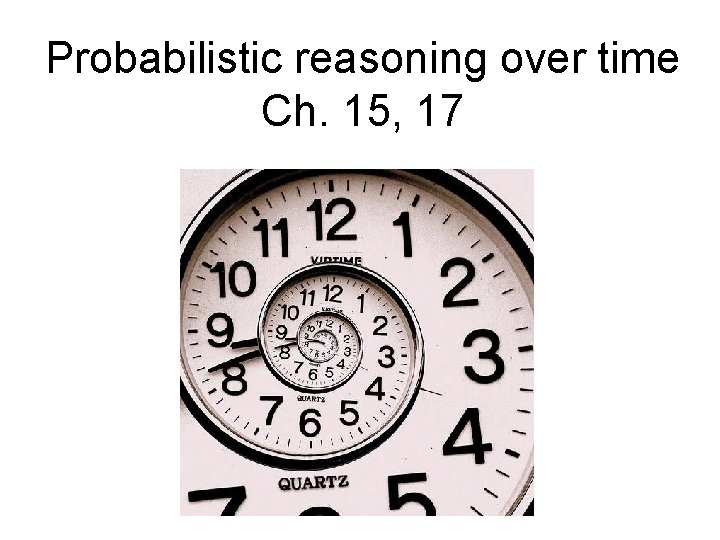Probabilistic reasoning over time Ch. 15, 17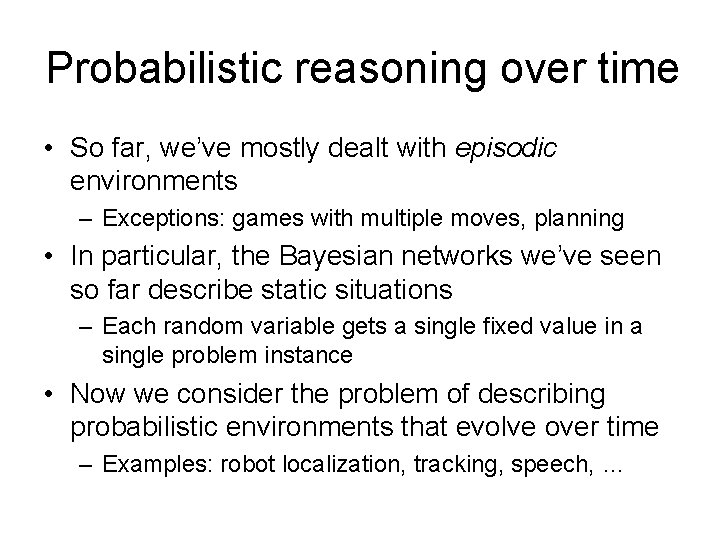Probabilistic reasoning over time • So far, we’ve mostly dealt with episodic environments – Exceptions: games with multiple moves, planning • In particular, the Bayesian networks we’ve seen so far describe static situations – Each random variable gets a single fixed value in a single problem instance • Now we consider the problem of describing probabilistic environments that evolve over time – Examples: robot localization, tracking, speech, …Hidden Markov Models • At each time slice t, the state of the world is described by an unobservable variable Xt and an observable evidence variable Et • Transition model: distribution over the current state given the whole past history: P(Xt | X 0, …, Xt-1) = P(Xt | X 0: t-1) • Observation model: P(Et | X 0: t, E 1: t-1) X 0 X 1 X 2 E 1 E 2 … Xt-1 Xt Et-1 EtHidden Markov Models • Markov assumption (first order) – The current state is conditionally independent of all the other states given the state in the previous time step – What does P(Xt | X 0: t-1) simplify to? P(Xt | X 0: t-1) = P(Xt | Xt-1) • Markov assumption for observations – The evidence at time t depends only on the state at time t – What does P(Et | X 0: t, E 1: t-1) simplify to? P(Et | X 0: t, E 1: t-1) = P(Et | Xt) X 0 X 1 X 2 E 1 E 2 … Xt-1 Xt Et-1 Et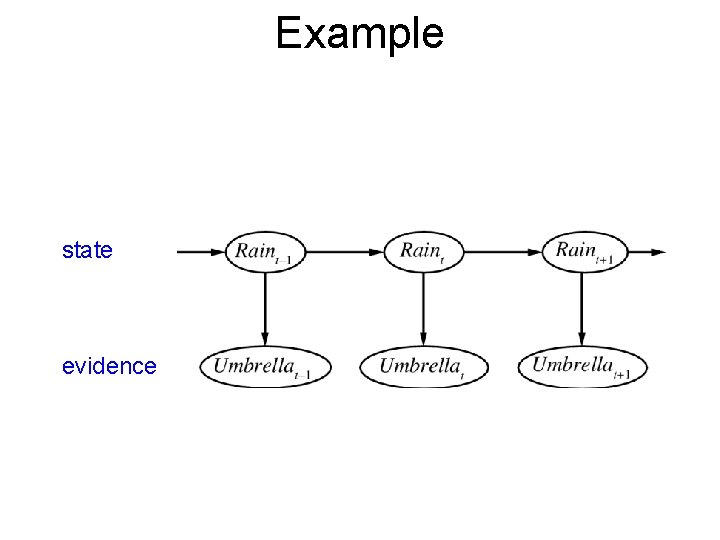Example state evidence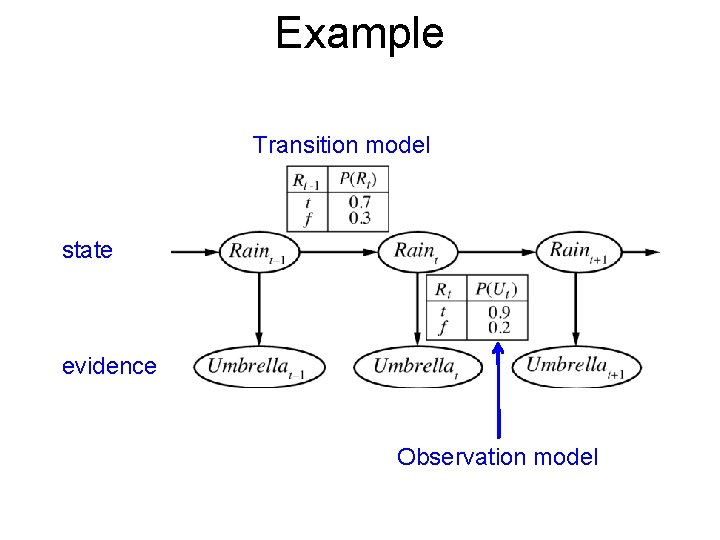Example Transition model state evidence Observation modelAn alternative visualization U=T: 0. 9 U=F: 0. 1 0. 3 0. 7 R=T 0. 7 Transition probabilities R=F 0. 3 Rt = T Rt = F Rt-1 = T 0. 7 0. 3 Rt-1 = F 0. 3 0. 7 U=T: 0. 2 U=F: 0. 8 Observation (emission) probabilities Ut = T Ut = F Rt = T 0. 9 0. 1 Rt = F 0. 2 0. 8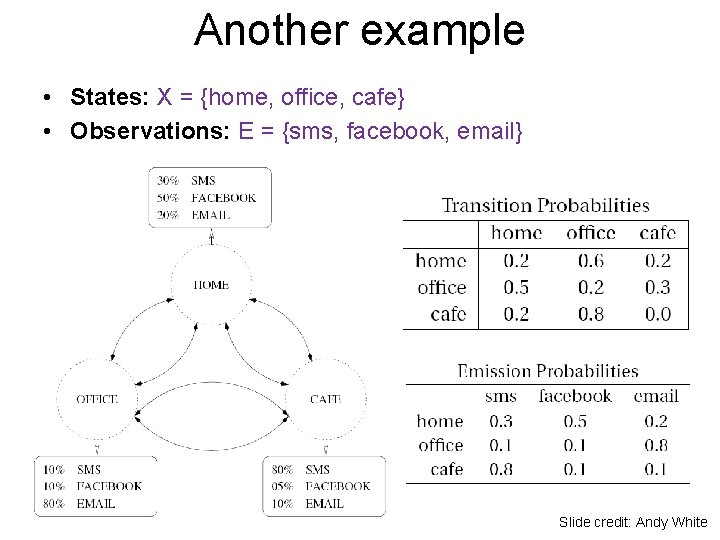Another example • States: X = {home, office, cafe} • Observations: E = {sms, facebook, email} Slide credit: Andy WhiteThe Joint Distribution • Transition model: P(Xt | X 0: t-1) = P(Xt | Xt-1) • Observation model: P(Et | X 0: t, E 1: t-1) = P(Et | Xt) • How do we compute the full joint P(X 0: t, E 1: t)? X 0 X 1 X 2 E 1 E 2 … Xt-1 Xt Et-1 EtReview: Bayes net inference • Computational complexity • Special cases • Parameter learning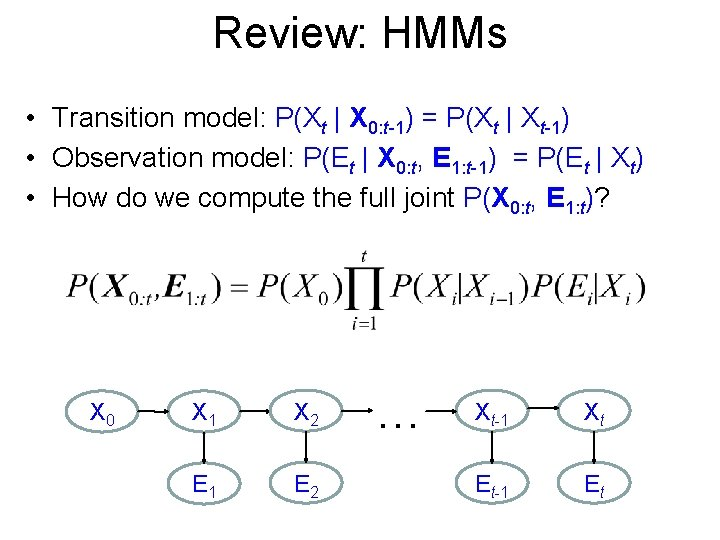Review: HMMs • Transition model: P(Xt | X 0: t-1) = P(Xt | Xt-1) • Observation model: P(Et | X 0: t, E 1: t-1) = P(Et | Xt) • How do we compute the full joint P(X 0: t, E 1: t)? X 0 X 1 X 2 E 1 E 2 … Xt-1 Xt Et-1 Et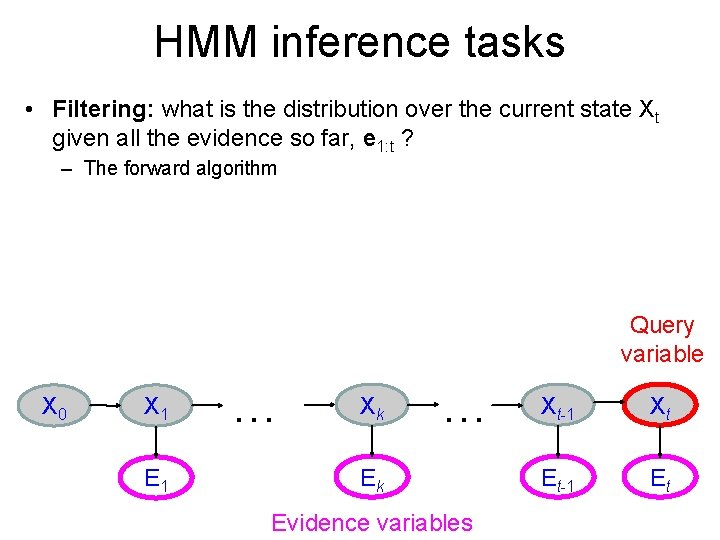HMM inference tasks • Filtering: what is the distribution over the current state Xt given all the evidence so far, e 1: t ? – The forward algorithm Query variable X 0 X 1 E 1 … Xk … Ek Evidence variables Xt-1 Xt Et-1 Et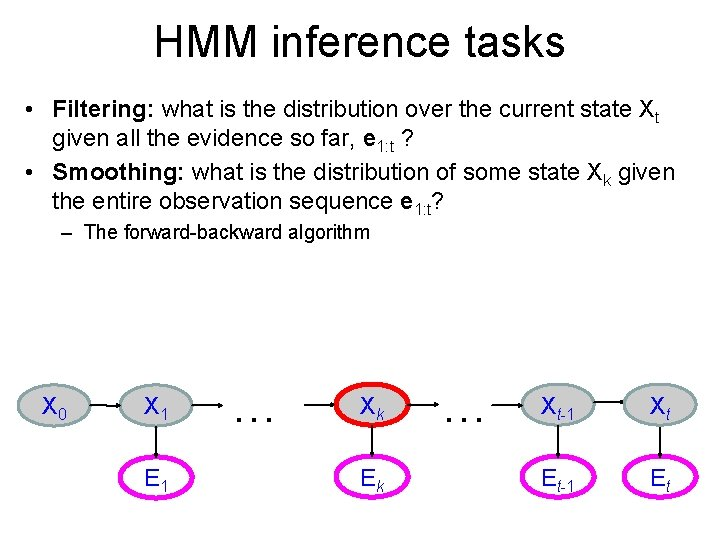HMM inference tasks • Filtering: what is the distribution over the current state Xt given all the evidence so far, e 1: t ? • Smoothing: what is the distribution of some state Xk given the entire observation sequence e 1: t? – The forward-backward algorithm X 0 X 1 E 1 … Xk Ek … Xt-1 Xt Et-1 EtHMM inference tasks • Filtering: what is the distribution over the current state Xt given all the evidence so far, e 1: t ? • Smoothing: what is the distribution of some state Xk given the entire observation sequence e 1: t? • Evaluation: compute the probability of a given observation sequence e 1: t X 0 X 1 E 1 … Xk Ek … Xt-1 Xt Et-1 EtHMM inference tasks • Filtering: what is the distribution over the current state Xt given all the evidence so far, e 1: t • Smoothing: what is the distribution of some state Xk given the entire observation sequence e 1: t? • Evaluation: compute the probability of a given observation sequence e 1: t • Decoding: what is the most likely state sequence X 0: t given the observation sequence e 1: t? – The Viterbi algorithm X 0 X 1 E 1 … Xk Ek … Xt-1 Xt Et-1 Et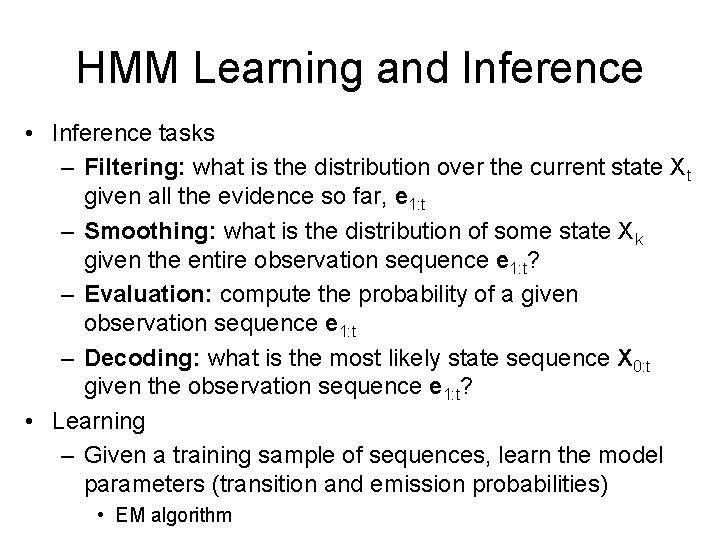HMM Learning and Inference • Inference tasks – Filtering: what is the distribution over the current state Xt given all the evidence so far, e 1: t – Smoothing: what is the distribution of some state Xk given the entire observation sequence e 1: t? – Evaluation: compute the probability of a given observation sequence e 1: t – Decoding: what is the most likely state sequence X 0: t given the observation sequence e 1: t? • Learning – Given a training sample of sequences, learn the model parameters (transition and emission probabilities) • EM algorithm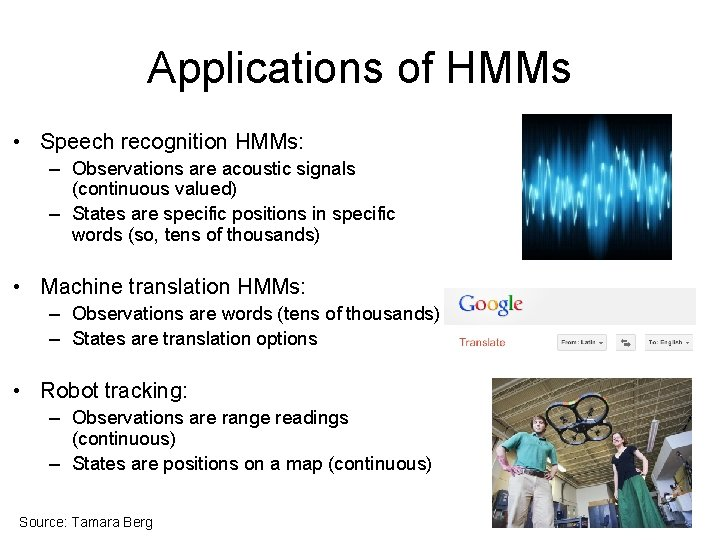Applications of HMMs • Speech recognition HMMs: – Observations are acoustic signals (continuous valued) – States are specific positions in specific words (so, tens of thousands) • Machine translation HMMs: – Observations are words (tens of thousands) – States are translation options • Robot tracking: – Observations are range readings (continuous) – States are positions on a map (continuous) Source: Tamara Berg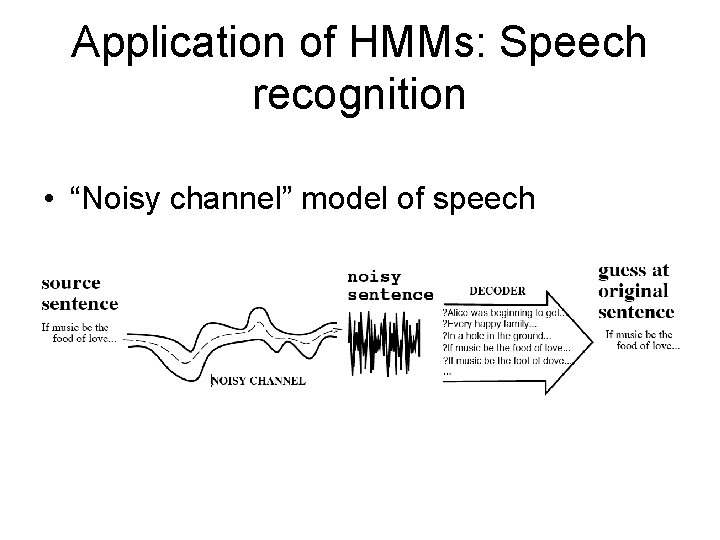Application of HMMs: Speech recognition • “Noisy channel” model of speechFrequency Acoustic wave form Sampled at 8 KHz, quantized to 8 -12 bits Spectrogram Frame (10 ms or 80 samples) Feature vector ~39 dim. Amplitude Speech feature extraction Time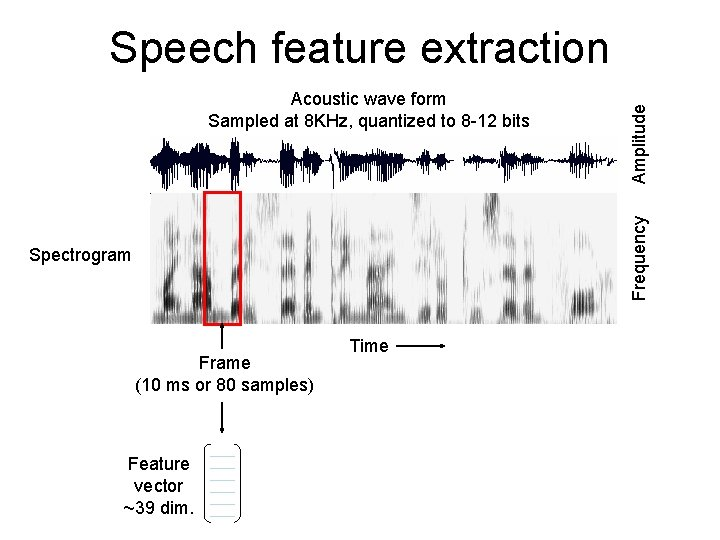Frequency Acoustic wave form Sampled at 8 KHz, quantized to 8 -12 bits Amplitude Speech feature extraction Spectrogram Frame (10 ms or 80 samples) Feature vector ~39 dim. TimePhonetic model • Phones: speech sounds • Phonemes: groups of speech sounds that have a unique meaning/function in a language (e. g. , there are several different ways to pronounce “t”)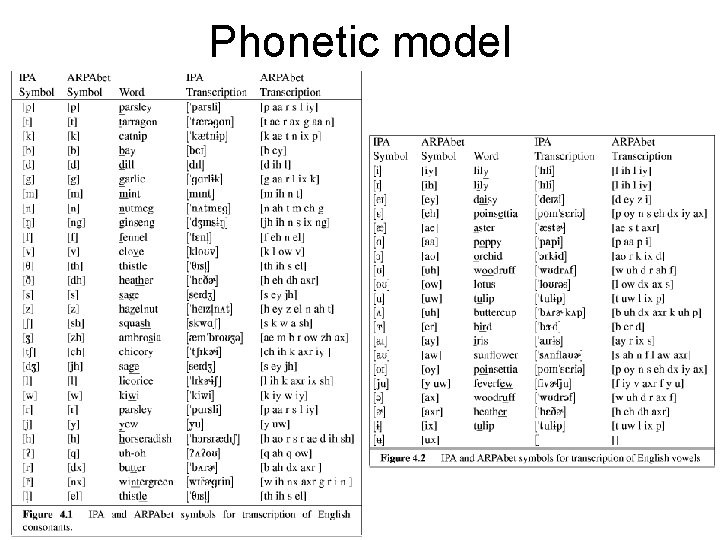Phonetic model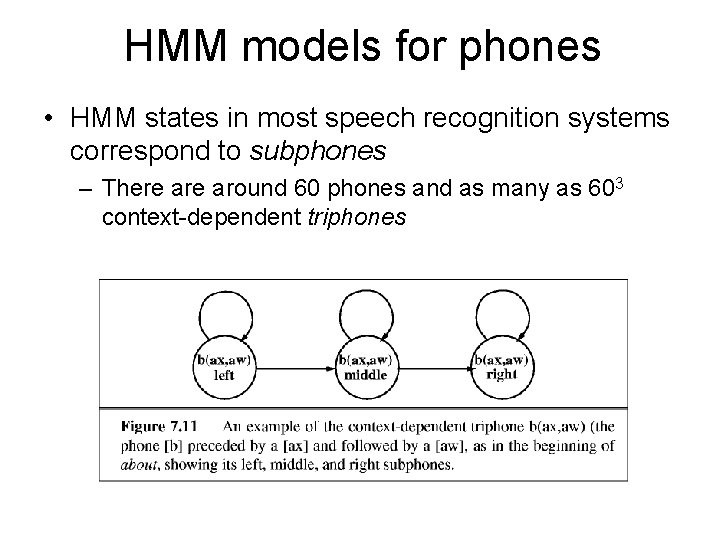HMM models for phones • HMM states in most speech recognition systems correspond to subphones – There around 60 phones and as many as 603 context-dependent triphonesHMM models for wordsPutting words together • Given a sequence of acoustic features, how do we find the corresponding word sequence?Decoding with the Viterbi algorithm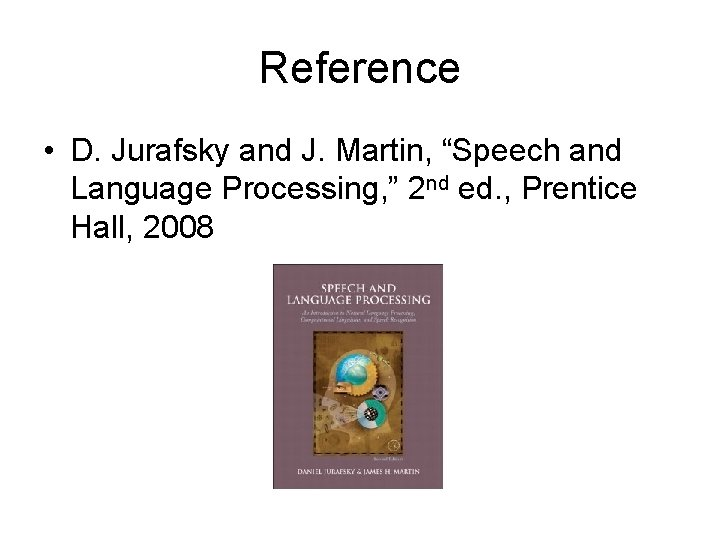Reference • D. Jurafsky and J. Martin, “Speech and Language Processing, ” 2 nd ed. , Prentice Hall, 2008